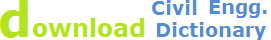Geodetic Survey, classification and types of triangulation system

# How to Trigonometric Surveying - Geodetic Survey

## Definition:

Geodetic or trigonometric surveying takes into account the curvature of earth. Since very extensive areas and very large distances are involved, curvature of the earth has to be consodered. In geodic surveying highly refined instruments and methods are used for taking measurements. Geodetic work is usually undertaken by a state agency e.g. Survey of Pakistan. It consists of:

1. Triangulation
2. Precise Leveling

## Object:

The object of geodetic surveying is to accurately determine the relative position of a sys of widely separated pts (stations) on the surface of earth and also their absolute positions.

Also Read:Errors in Levelling |Survey Tools Maintenance |Highway Curves Survey

Relative positions are determined in terms of azimuths and length f lines joining them. Absolute positions are determined in terms of latitude and longitudes and elevations above mean sea laves. The methods employed in geodetic surveying are:

1. Triangulation (most accurate but expensive)
2. Precise traverse (inferior and used when triangulation is physically impossible or very expensive) e.g. Densely wooded country.

## Triangulation:

It is based on the trigonometry proposition that of one side and three angles be computed by the application of since rule. In this method suitable points called triangulation stations are selected and established throughout the area to be surveyed. The stations may be connected by a series of triangles or a chain of quadrilaterals as shown.### Baseline:

Whose length is measured these stations form the vertices of a series mutually co nnected, triangles the complete figure being called ‘Triangulation system’. In this system of triangles one line say ‘AB’ and all the angles are measured with greatest care and lengths of all the remaining line in the system are then computed. For checking both the fieldwork and computation another line say GH is very accurately measured at the end of the system. The line whose length is actually measured is known as baseline or base and that measured for checking purpose is known as the check base.

### Triangulation Figures:

The geometric figures used in triangulation system are (i) Triangles (ii) Quadrilaterals (ii) Quadrilaterals, Pentagon, hexagons with centre angle. This arrangement although simple and economical but less accurate since the number of conditions involve in its adjustment is small.

1. Station adjustment ==> sum of angle is 180
2. Figure adjustment ==> sum of angles is 400 grad or 360Quadrilaterals pentagons or hexagonal with central stations. For very accurate work a chain of quadrilaterals may be used. There is no station at the intersection of diagonals. This system is most accurate since the number of conditions in its adjustments is much greater. To minimize the effect of small errors in measurement of angles the triangles hold be well shaped or well proportioned i.e. they should not have angle less than 30 or greater than 120.The best shape triangle is equilaterals triangle and best shape quadrilateral is square.

# Classification of Triangulation System

Triangulation system may be classified according to

1. Degree of accuracy required
2. Magnitude of work
1. ## Primary or 1st order Triangulation:

In primary triangulation very large areas (such as the whole country) are covered and the highest possible precision is secured. Well proportioned triangles, most refined instrument and methods of observations and computation are used.

Average triangle closure = 1 second
Max triangle closure = 3 second
Length of baseline = 5 to 20 km or more (3-10 miles)
Length of sides of triangle = 30 to 100 km or more (20-100 miles)
Degree of Accuracy = 1 in 500,000
Check on the base    = 1 in 25000.

1. ## Secondary or 2nd Order Triangulation

Within the primary triangles other points are fixed at closure intervals so as to form a secondary series of triangles. Which are comparatively smallare used, the instars and methods are not of the same at most refined.

Average triangle closure = 3sec

Max = 8 sec

= 2 to 5km [ 1-3 miles]

= 8 to 70 km [ 5-40 miles]

Degree of Accuracy = 1 in 50,000

Check on the base = 1 in 10,000

1. ## Tertiary or 3rd order Triangulation

Within the secondary &deltapts are established at short intervals to furnish horizontal control fro detail survey.

Averageclosure = 6 sec
= 12 sec
= 1 to 30 km [½ to ½]
= 1.5 to 10 km [less then a mile to 60 miles]

Degree of accuracy = 71 in 500

Check on base = 1 in 500

## Applications of Triangulation

1. The establishment of accurately located control points for surveys of large.
2. The accurate location of indirection work such as:
• Centre lines, terminal pts shafts for long tunnels
• Centers lines and abutment for bridges of longs spans.
• Complex highway interchanges.
3. The establishment of accurately located control pts in connection with aerial surveying.
4. Measurement of deformation of structure such as dams.

## Trilitration:

Because of the development of highly accurate electronic measuring devices, a triangulation system can be completely observed, computed and adjusted by measuring the lengths o the sides in the network. This procedure is known as trilitration. No horizontal angle need to be measured because the lengths of the sides are sufficient to permit both the horizontal angles and the positions of the stations to be computed.

The surveying solution technique of measuring only the side of triangle is called triplication.Site Map | Civil Engineering Training | Contact Us || © 2014 WebTechTix